"Margin+ (Margin Calculator)" App Analytics: ASO Keyword Monitoring | ASOTools

# "Margin+ (Margin Calculator)" App Analytics: ASO Keyword Monitoring | ASOTools

Are you doing Margin+ (Margin Calculator) app analytics for better app store ASO strategies? Whether Margin+ (Margin Calculator) in app store keep on top? What keyword tactics Margin+ (Margin Calculator) take to rank well in app store? Are app store keywords like "beverage margin calculator", "gross profit app", "gross profit margin calculator" matter in Margin+ (Margin Calculator) ranking?

Free ASO tools for Google Play & App Store, ASOTools will reveal the app store keywords secrets of Margin+ (Margin Calculator).

## App Analytics: Margin+ (Margin Calculator)

Margin+ (Margin Calculator)-Best Margin/Markup Calculator, published by James Spencer in app store, released on 2010-02-18, and last updated 2020-10-21. It belongs to Finance category, and Finance category is ranking 95 in app store. Currently, Margin+ (Margin Calculator) has 509 ratings, the average rating is 5. Additionally, it had - downloads and - revenue last month in app store worldwide, and the daily active users is -.

More Margin+ (Margin Calculator) info and other app analytics can be found freely in ASOTool.

## ASO Keyword Monitoring: Margin+ (Margin Calculator)

### 1.Margin+ (Margin Calculator) Top 10 App Store Keywords

 Keyword Search Volume KD Related Apps Margin+ (Margin Calculator)'s Rank beverage margin calculator 1 425 9 75 1 gross profit app - - 10 33 1 gross profit margin calculator 1 - 7 22 1 ieretail gp calculator 1 438 11 39 1 bisycalc retail margin calculator - - 9 75 1 margin calc pro - - 9 79 1 margins calculator 4 - 10 76 1 gross profit calculator 6 - 6 27 1 marginal cost calculator 2 - 8 27 1 imargin 1 430 11 172 1

App store keywords matter for ASO. If your app is similar to Margin+ (Margin Calculator), when you optimize app store keyword ranking, pay attention to the metrics above. ASOTools will show you all of them for free.

Obviously, Margin+ (Margin Calculator) is ranking for "beverage margin calculator", "gross profit app", "gross profit margin calculator", "ieretail gp calculator", "bisycalc retail margin calculator", "margin calc pro", "margins calculator", "gross profit calculator", "marginal cost calculator", and "imargin" these top 10 keywords in app store.

Also, you'll find Margin+ (Margin Calculator)'s position in app store search result. Margin+ (Margin Calculator) is ranking 1 for beverage margin calculator, ranking 1 for gross profit app, ranking 1 for gross profit margin calculator, and ranking 1 for ieretail gp calculator. For more Margin+ (Margin Calculator) keyword monitoring, please visit asotools.io.

### 2. Top 5 Keyword-Related Apps& App Analytics

• beverage margin calculator  Related Apps & App Analytics
 Apps Average Star RatingsMargin+ (Margin Calculator)-Best Margin/Markup Calculator 5 509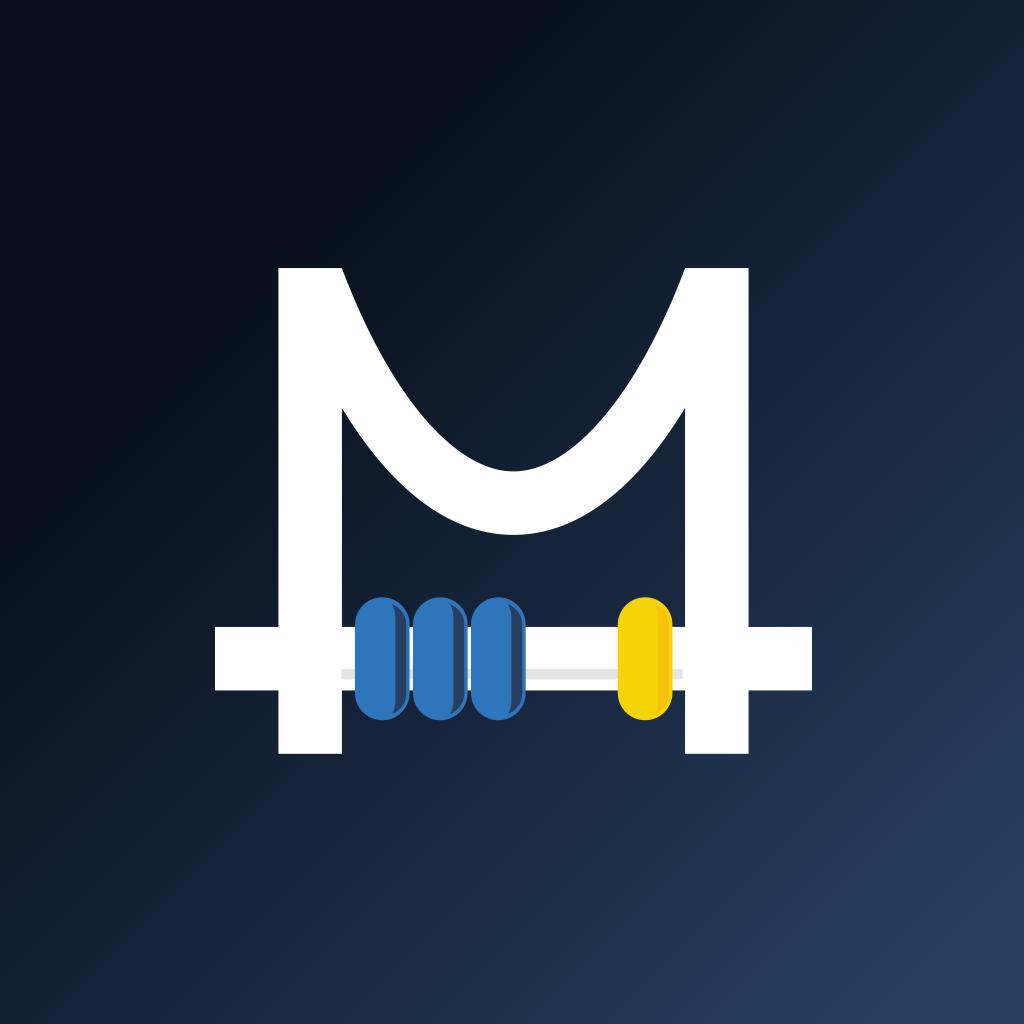Cost Margin Calculator 3 69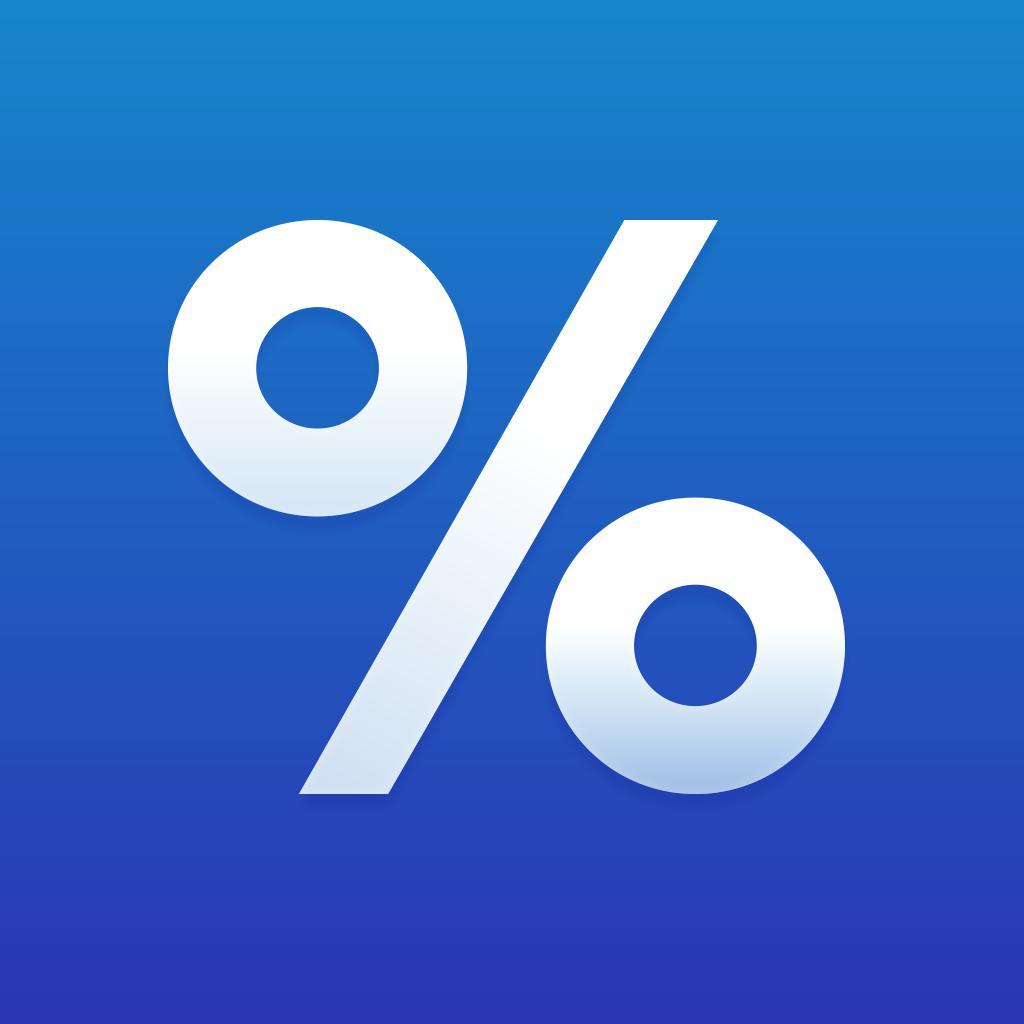Percentage Calculator ٞ-Calculate percents & fractions 4 3667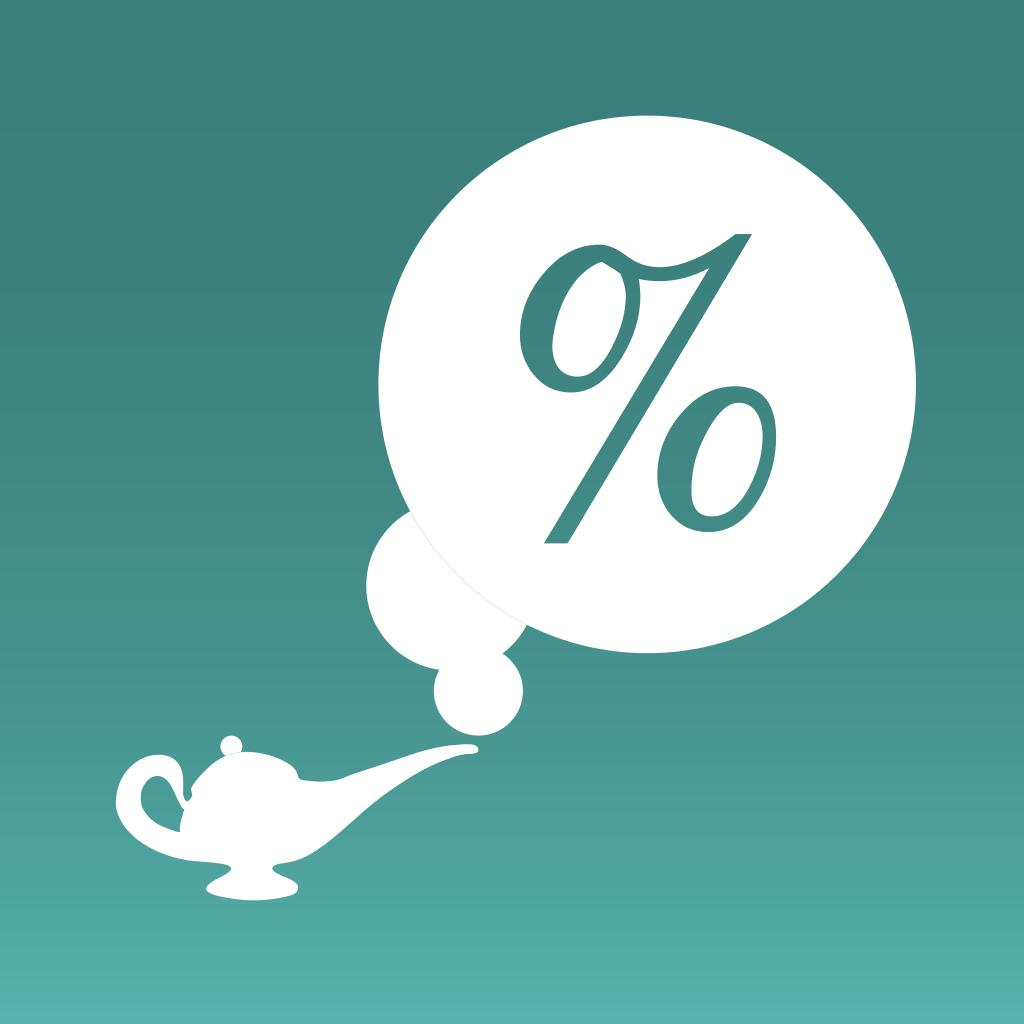Mar-Genie Margin Calculator 4 21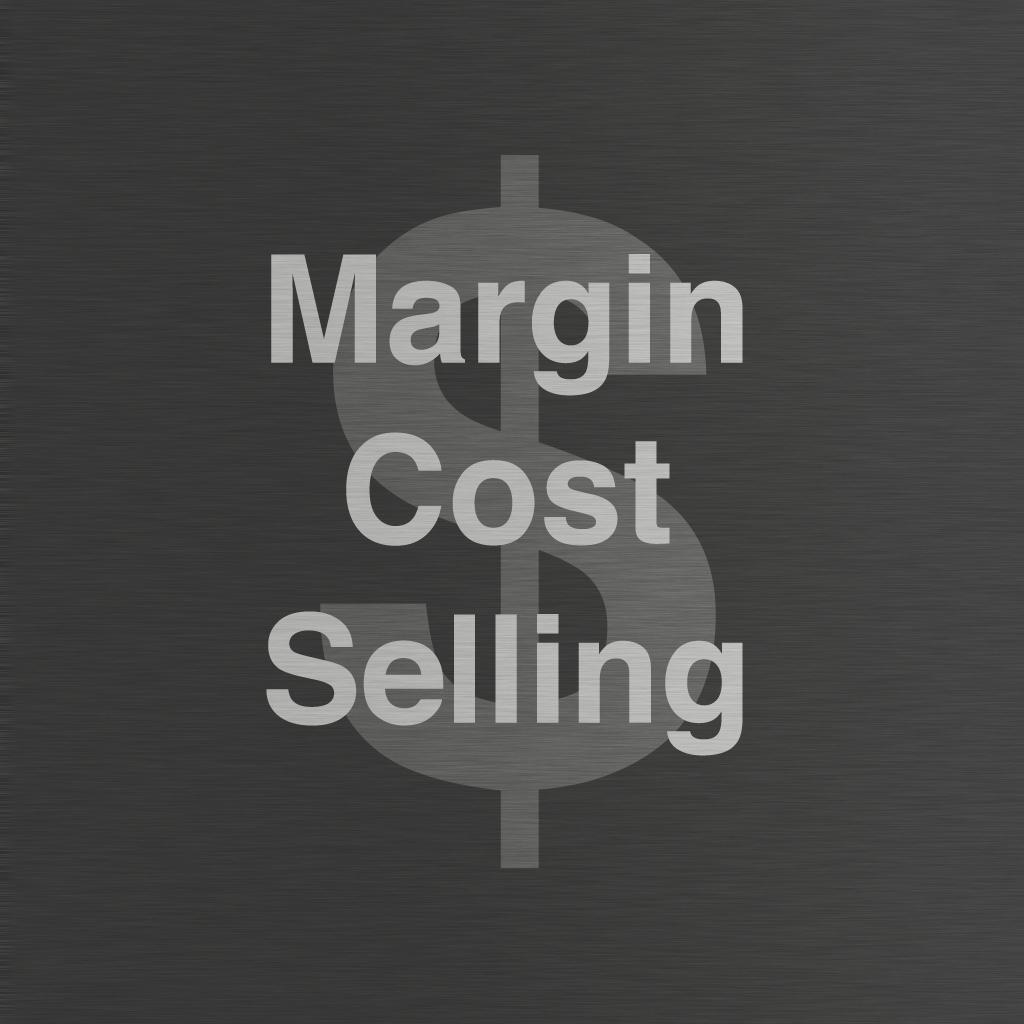Margin and Markup Calculator 3 2

"beverage margin calculator" has 75 apps. Top 5 are Margin+ (Margin Calculator), Cost Margin Calculator, Percentage Calculator ٞ, Mar-Genie Margin Calculator, and Margin and Markup Calculator.

• gross profit app  Related Apps & App Analytics
 Apps Average Star RatingsMargin+ (Margin Calculator)-Best Margin/Markup Calculator 5 509Cost Margin Calculator 3 69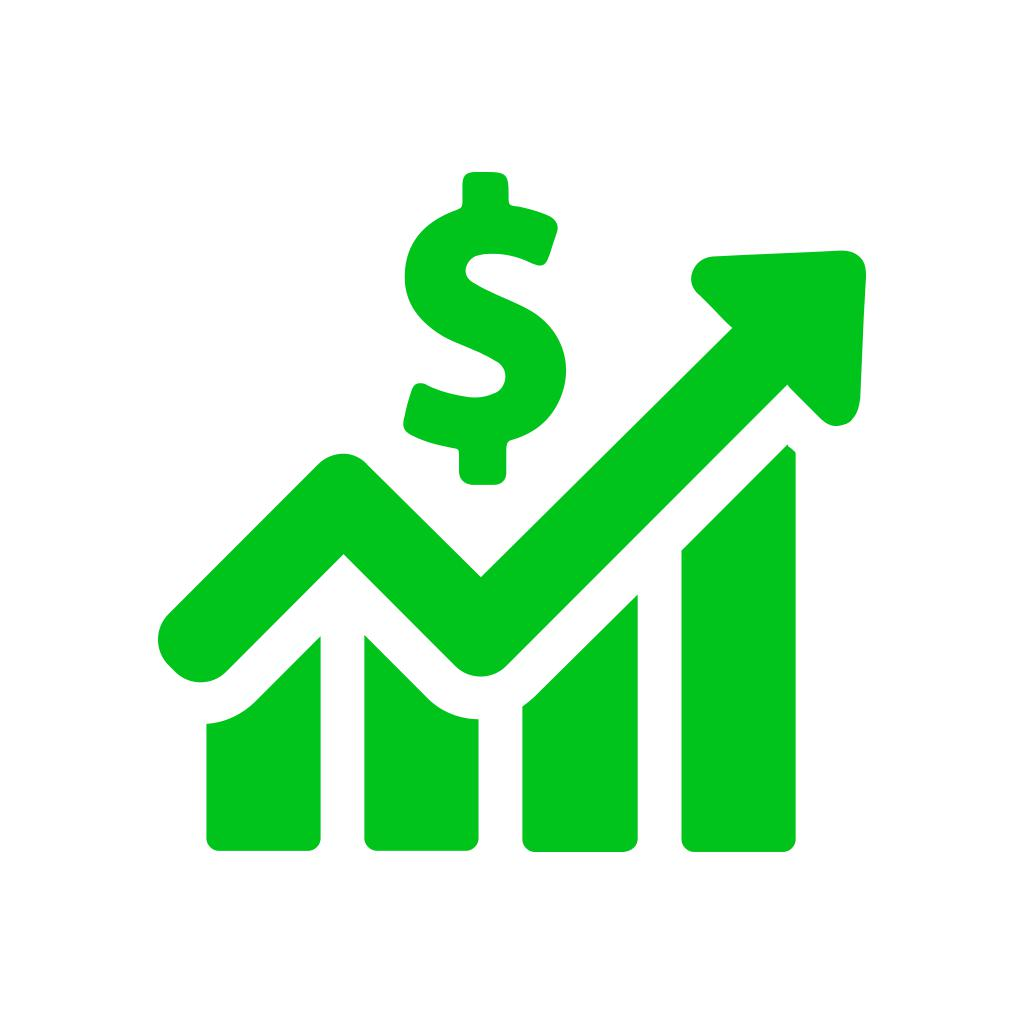Profit Finder - Fee Calculator-For eBay, Amazon, and PayPal 4 123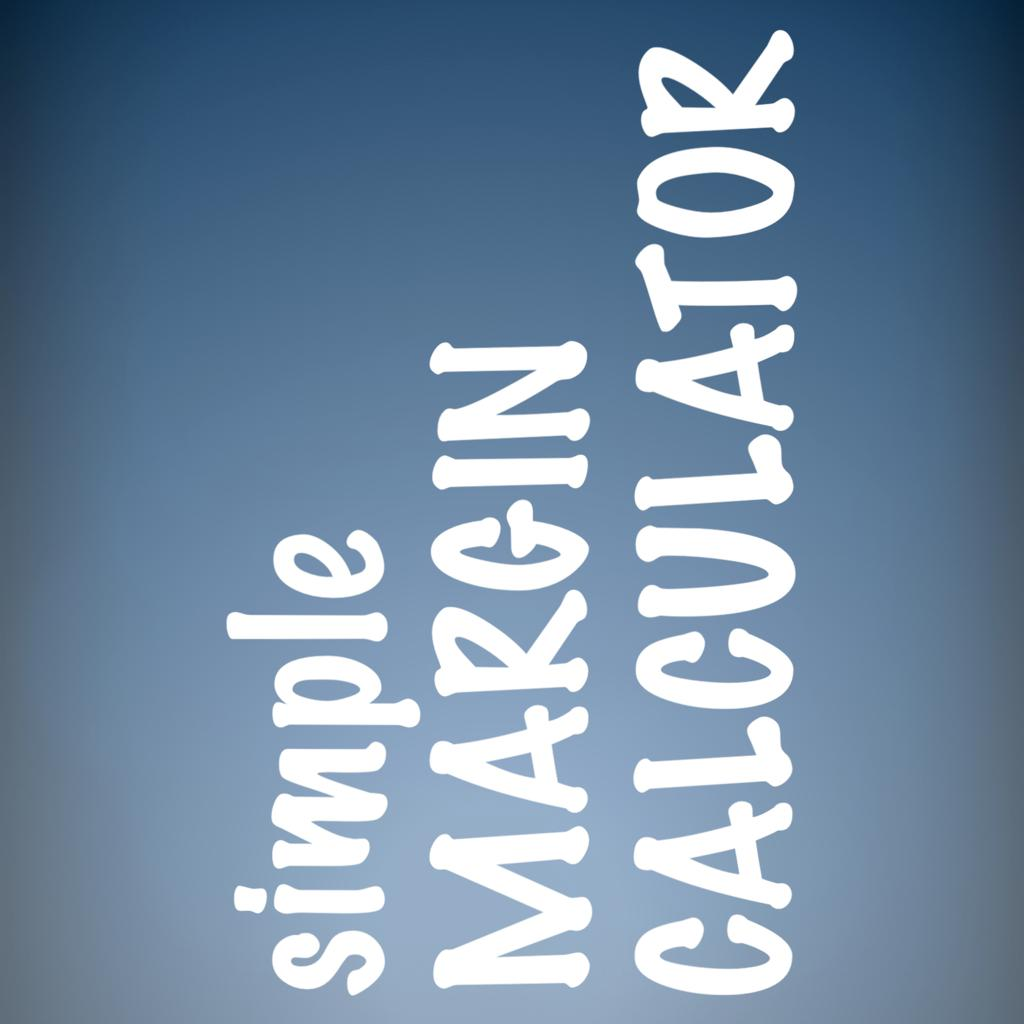Margin/Selling/Cost Calculator-SCPM - Simple Price Calculator 4 101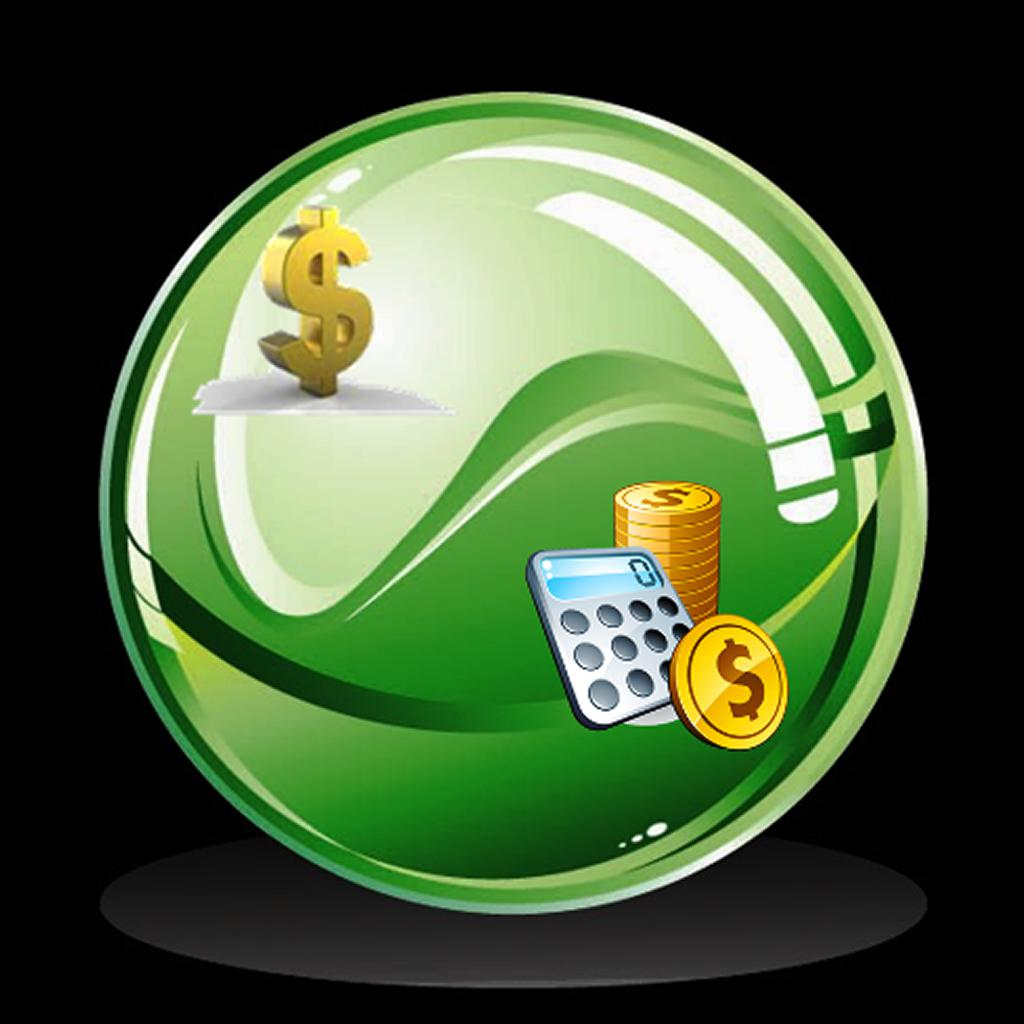Profit Story 4 10

"gross profit app" has 33 related apps in total. Top 5 are Margin+ (Margin Calculator), Cost Margin Calculator, Profit Finder - Fee Calculator, Margin/Selling/Cost Calculator, and Profit Story.

• gross profit margin calculator  Related Apps & App Analytics
 Apps Average Star RatingsMargin+ (Margin Calculator)-Best Margin/Markup Calculator 5 509Cost Margin Calculator 3 69Margin/Selling/Cost Calculator-SCPM - Simple Price Calculator 4 101Profit Finder - Fee Calculator-For eBay, Amazon, and PayPal 4 123ieRetail Calculator-Gross profit, margin, markup. - -

"gross profit margin calculator" has 22 related apps in app store. Top 5 are Margin+ (Margin Calculator), Cost Margin Calculator, Margin/Selling/Cost Calculator, Profit Finder - Fee Calculator, and ieRetail Calculator.

• ieretail gp calculator  Related Apps & App Analytics
 Apps Average Star Ratings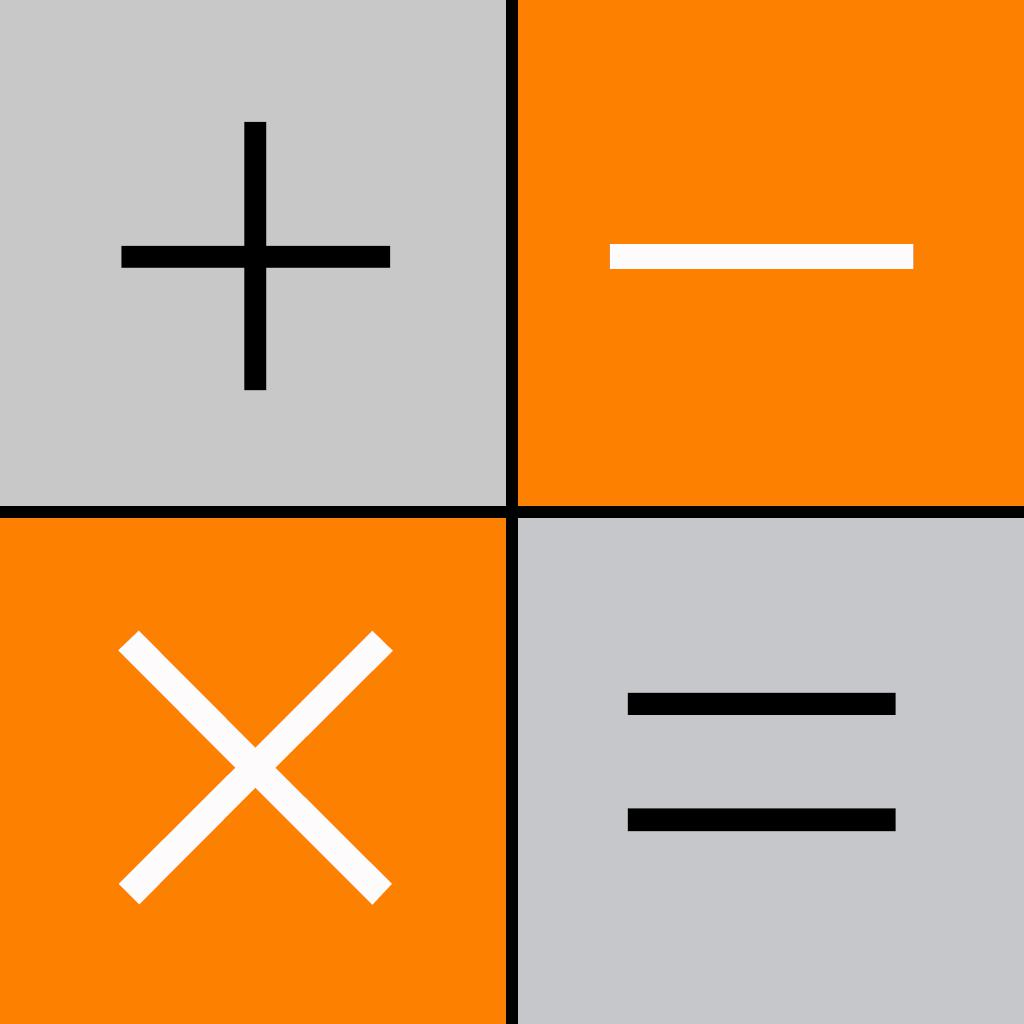Privault-Hide Private Photo.s Video.s HiCalculator 4 61212Margin+ (Margin Calculator)-Best Margin/Markup Calculator 5 509Cost Margin Calculator 3 69Profit Finder - Fee Calculator-For eBay, Amazon, and PayPal 4 123Margin/Selling/Cost Calculator-SCPM - Simple Price Calculator 4 101

"ieretail gp calculator" has 39 related apps. Top 5 apps are Privault-Hide Private Photo.s Video.s HiCalculator, Margin+ (Margin Calculator), Cost Margin Calculator, Profit Finder - Fee Calculator, and Margin/Selling/Cost Calculator.

• bisycalc retail margin calculator  Related Apps & App Analytics
 Apps Average Star RatingsCost Margin Calculator 3 69Margin+ (Margin Calculator)-Best Margin/Markup Calculator 5 509Percentage Calculator ٞ-Calculate percents & fractions 4 3667Mar-Genie Margin Calculator 4 21Margin and Markup Calculator 3 2

75 apps are ranking for "bisycalc retail margin calculator" in app store. Top 5 apps are Cost Margin Calculator, Margin+ (Margin Calculator), Percentage Calculator ٞ, Mar-Genie Margin Calculator, and Margin and Markup Calculator.

As you can see, Margin+ (Margin Calculator), Margin+ (Margin Calculator), and Cost Margin Calculator are ranking for beverage margin calculator, also, Margin+ (Margin Calculator), Margin+ (Margin Calculator), Cost Margin Calculator and Margin/Selling/Cost Calculator are all competing the keyword gross profit app.

Before promoting your apps in app store, you'd better perform app analytics for better ASO tactics, and ASOTools, free ASO tools will help you with App store keywords optimization.